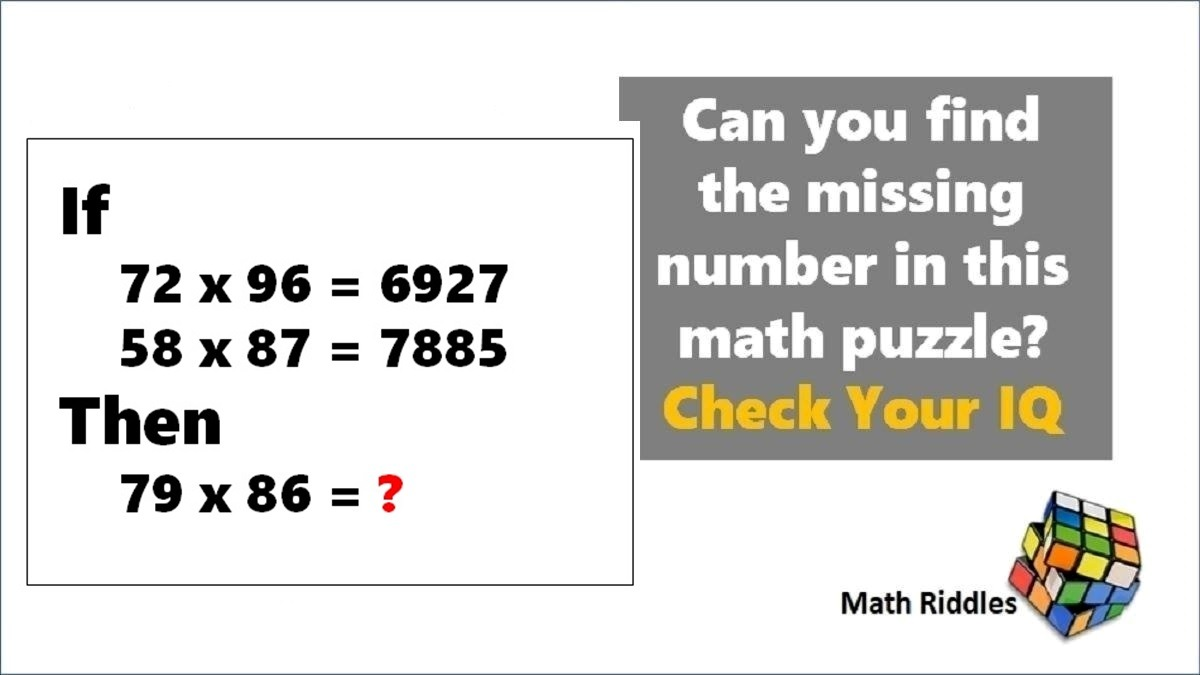# Math Riddles: Only 1 out 5 People Can Solve These 5-Minute Maths Puzzles

Math Riddles Challenge: You are 100% genius if you can find the logic and solve this mathematics puzzle in less than 1 minute each?Math Riddles: Only 1 out 5 People Can Solve These 5-Minute Maths Puzzles

Math Riddles: Check out these 5-minute mathematics puzzles that only 1% genius people can find the solution. Can you solve them correctly in less than 1 minute each? You are a 100% genius if you can solve these math problems. These problems will aid you in preparation for government exams, banking, civil services aptitude, and logical reasoning test. Let us begin some brain exercises.

## Solution

### Maths Logic Puzzle #1

Explanation:

If you notice carefully, you will the products in the question statement are written in backwards. See below:

Similarly, we shall figure the answer to the 79 x 86 = ? in the puzzle.

### Maths Logic Puzzle #2

Explanation:

The logic in this mathematics puzzle is replacing the mathematical operations as stated in the question statement.

After replacing all the operations as per the question as below:

We shall apply BODMAS to the order of solving this equation.

6 + 36 ÷ 4 - 2 × 2 = ?

Step 1: Division => 6 + 9 – 2 x 2

Step 2: Multiplication => 6 + 9 – 4

Step 3: Addition => 15 – 4

Step 4: Subtraction => 11

## Tell us in comments: Did you solve these mathematics puzzles in less than 3 minutes each?

Check out more math puzzles!

Math Riddles: 5-Minute Maths Puzzles, Find the Answers in Less than 1 Minute

Also Read: Math Riddles: 5-Minute Mathematics Puzzles, 99% Failed! Can You Solve Them?

Also Read: Math Riddles: Only 1% Genius Can Solve These Tricky Mathematics Puzzles in 3 Minutes

Also Read: Math Riddles: Only 1% Can Solve This Math Crossword Puzzle, Difficulty Level Hard

Get the latest General Knowledge and Current Affairs from all over India and world for all competitive exams.
खेलें हर किस्म के रोमांच से भरपूर गेम्स सिर्फ़ जागरण प्ले पर